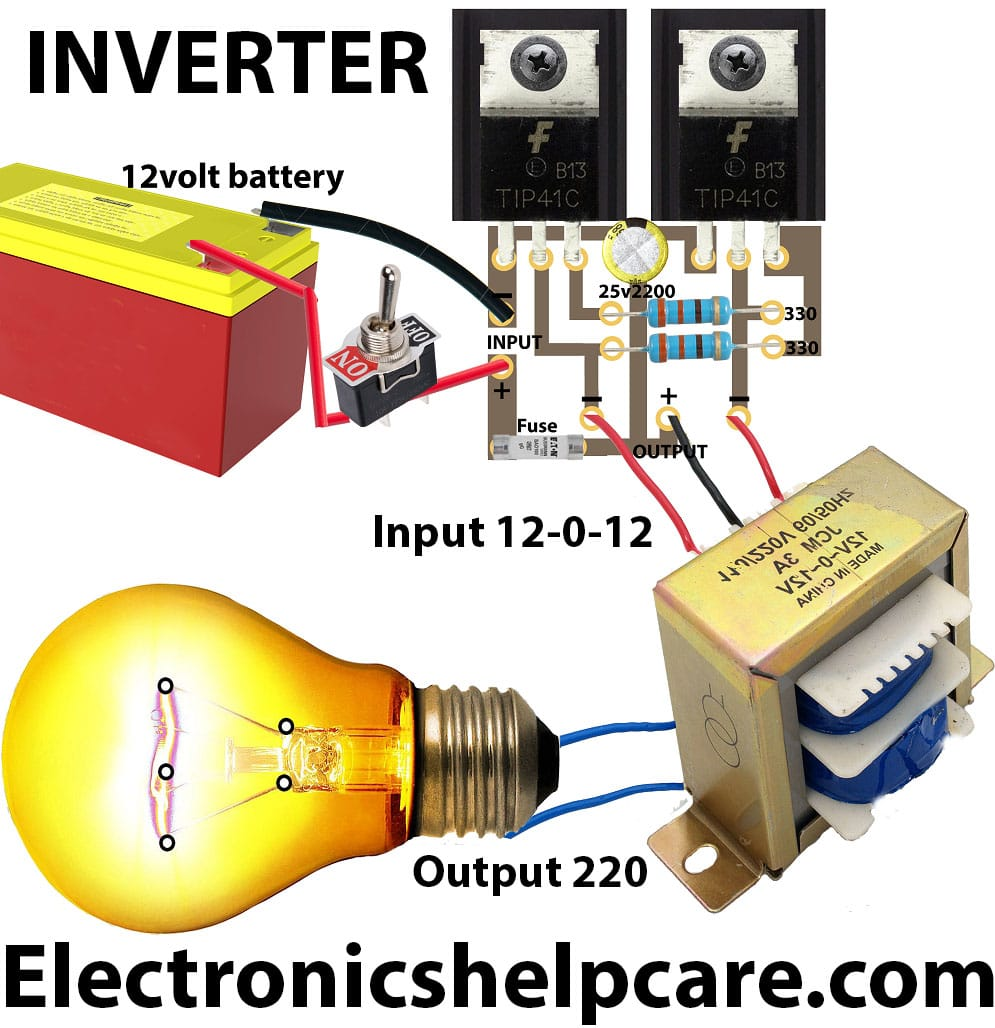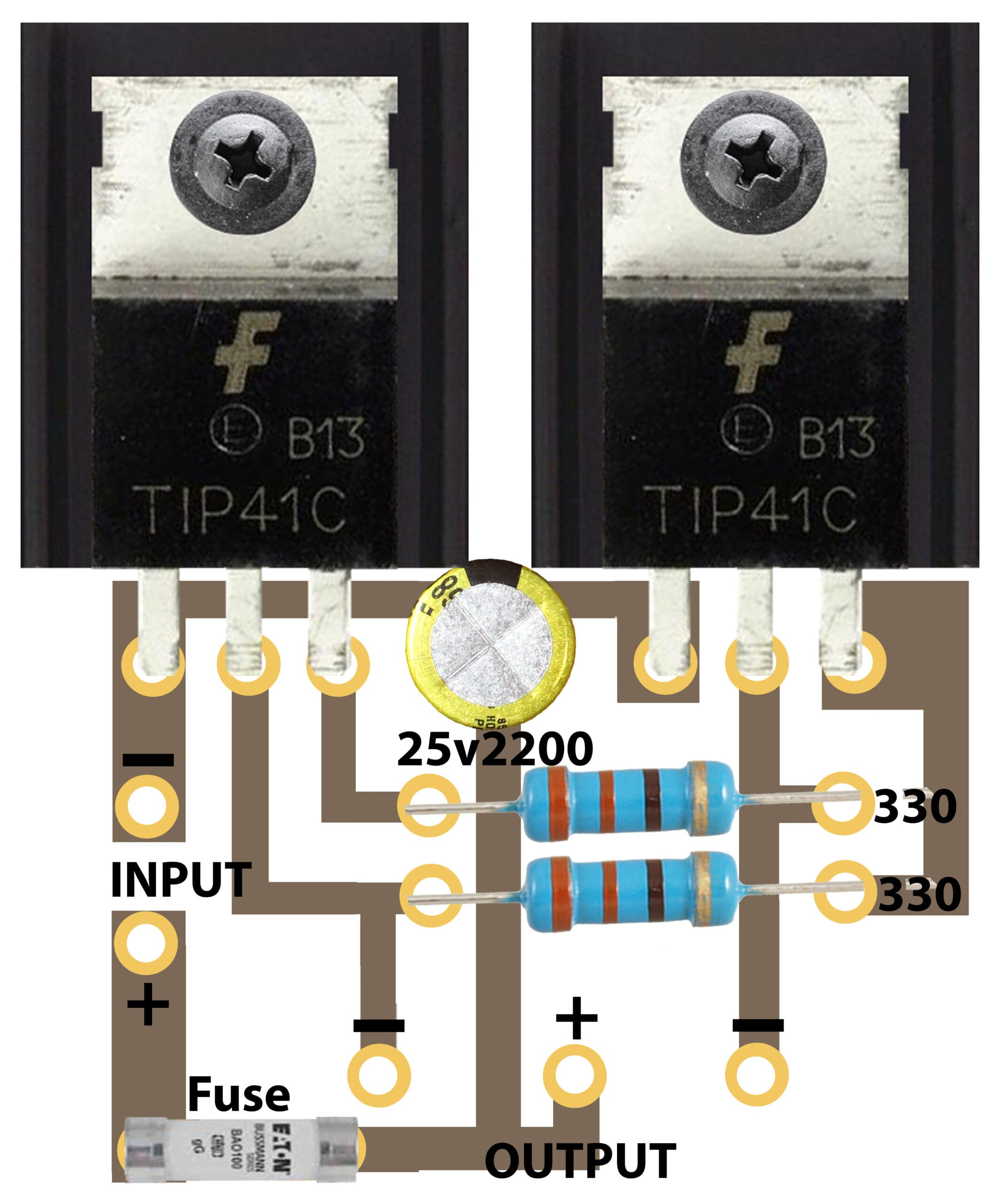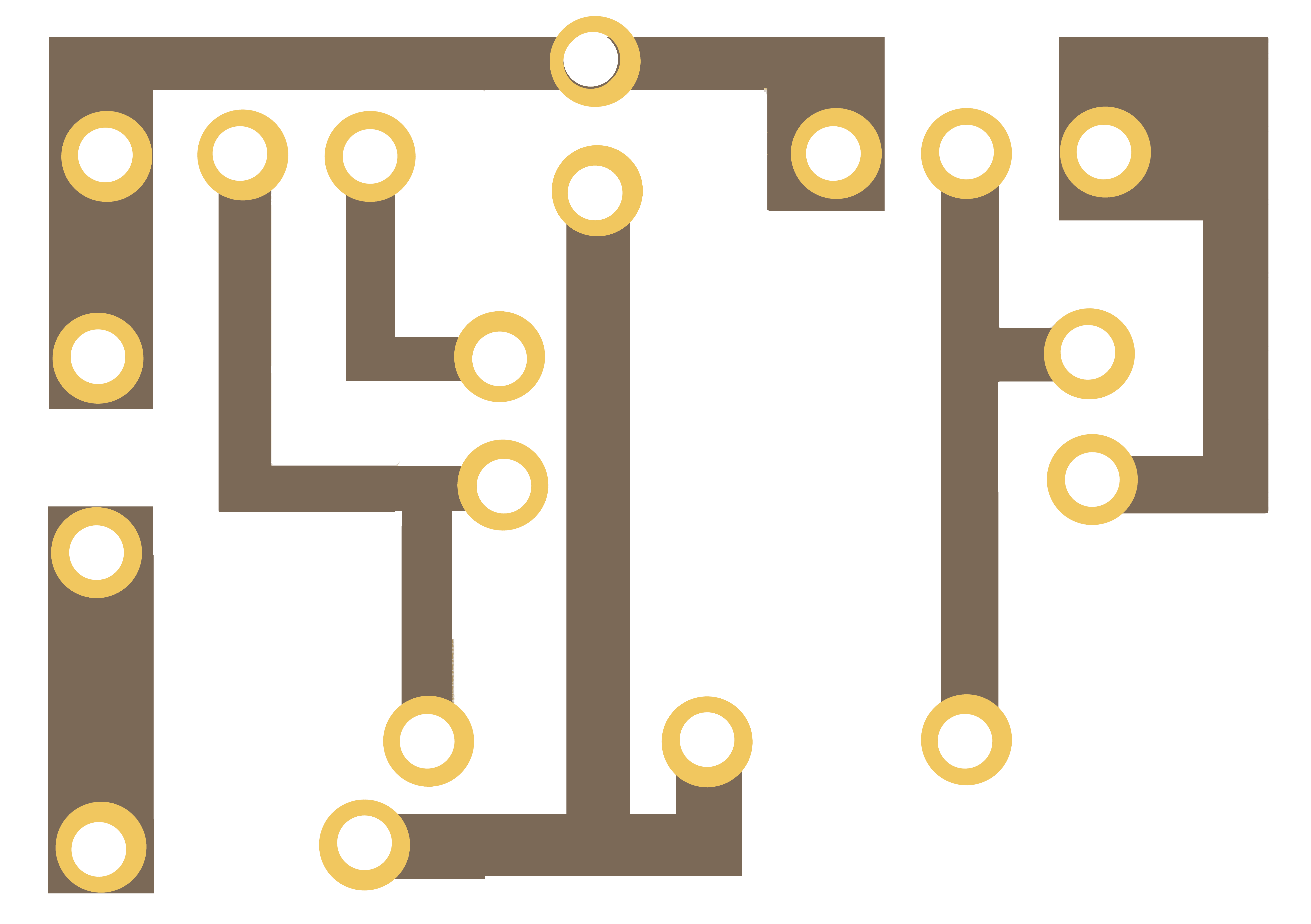Breaking News

# how to make 12 volt inverter

## how to make 12 volt inverter:

How do make 12 volts to 220 volts Inverter Circuit diagrams? this is an easy simple inverter circuit diagram. it’s can make a maximum of 50 watts using 2 transistors and this type of transformer. It’s not a real inverter. this circuit is for learning people. it’s a technology of an inverter.Here we use a normal step-down transformer. this transformer is a 3-amper transformer. we know that volt X amperes= Watts. So, 12X3= 36 watts. this transformer can make 36 watts only. here in this circuit used 2 Tip41c transistors (we can use any PNP  transistors). and used 2 resistors are 330 ohms and 2 watts.Circuit diagram12-volt inverter Circuit

Our channel videotransistor circuit diagram of 2sa1943 and 2sc5200

Thanks a lot to be with us. If you want another post then please visit our website.

We have another post for you. like as repairing amplifier,